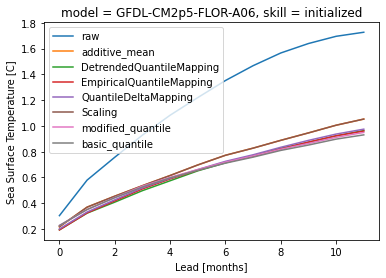You can run this notebook in a live sessionor view it on Github.

# Bias Removal#

Climate models can have biases relative to different verification datasets. Commonly, biases are removed by postprocessing before verification of forecasting skill . `climpred` provides convenience functions to do so.

The select from following methods by specifying `how` in `HindcastEnsemble.remove_bias()`:

• `how="additive_mean"`: correcting the mean forecast additively

• `how="multiplicative_mean"`: correcting the mean forecast multiplicatively

• `how="multiplicative_std"`: correcting the standard deviation multiplicatively

Wrapped from the github package `bias_correction`:

• `how="modified_quantile"`: Reference

• `how="basic_quantile"`: Reference

• `how="gamma_mapping"`: Reference

• `how="normal_mapping"`: Reference

Wrapped from `xlim.sdba`:

```# linting
```
```import climpred
import xarray as xr
import matplotlib.pyplot as plt
from climpred import HindcastEnsemble
```
```initialized = climpred.tutorial.load_dataset("NMME_hindcast_Nino34_sst")
v = "sst"
```
```hindcast = HindcastEnsemble(
initialized.sel(model="GFDL-CM2p5-FLOR-A06")
hindcast.plot()
```
```/Users/aaron.spring/Coding/climpred/climpred/checks.py:202: UserWarning: Did not find dimension "init", but renamed dimension S with CF-complying standard_name "forecast_reference_time" to init.
warnings.warn(
/Users/aaron.spring/Coding/climpred/climpred/checks.py:202: UserWarning: Did not find dimension "member", but renamed dimension M with CF-complying standard_name "realization" to member.
warnings.warn(
/Users/aaron.spring/Coding/climpred/climpred/checks.py:202: UserWarning: Did not find dimension "lead", but renamed dimension L with CF-complying standard_name "forecast_period" to lead.
warnings.warn(
```
```<AxesSubplot:xlabel='validity time', ylabel='Sea Surface Temperature\n[Celsius_scale]'>
```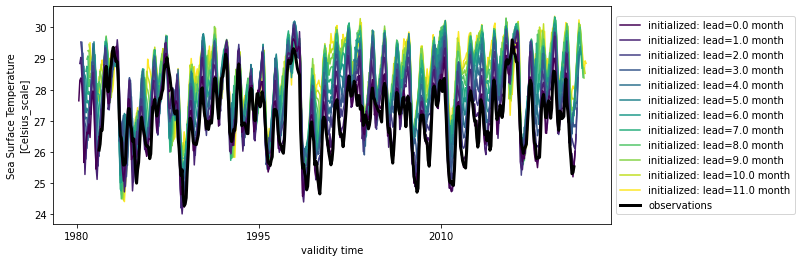Typically, bias depends on lead-time and therefore should therefore also be removed depending on `lead`.

```bias = hindcast.verify(
)

bias[v].plot()
```
```<matplotlib.collections.QuadMesh at 0x143700d60>
```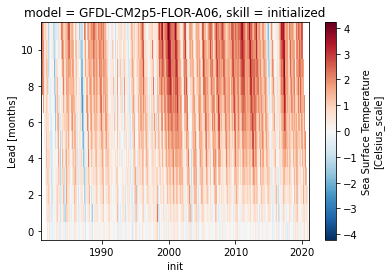```# group bias by seasonality
seasonality = climpred.options.OPTIONS["seasonality"]
seasonality
```
```'month'
```
```bias.groupby(f"init.{seasonality}").mean()[v].plot()
```
```<matplotlib.collections.QuadMesh at 0x1436fbd90>
```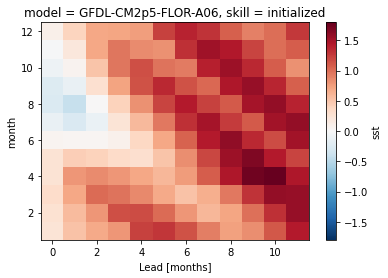An initial warm bias develops into a cold bias, especially in winter.

# `train_test_split`#

Risbey et al.  demonstrate how important a clean separation of a `train` and a `test` period is for bias reduction.

Implemented `train_test_split`s in `climpred`:

• `unfair`: completely overlapping `train` and `test` (climpred default)

• `unfair-cv`: overlapping `train` and `test` except for current `init`, which is left out (set `cv="LOO"`)

• `fair`: no overlap between `train` and `test` (recommended)

```metric_kwargs = dict(
metric="rmse", alignment="same_verifs", dim="init", comparison="e2o", skipna=True
)
```
```# fair calculates bias for train_time/train_init and drops these data from hindcast
hindcast.remove_bias(
alignment=metric_kwargs["alignment"],
train_test_split="fair",
train_time=slice("1982", "1998"),
).plot()
```
```<AxesSubplot:xlabel='validity time', ylabel='Sea Surface Temperature\n[Celsius_scale]'>
```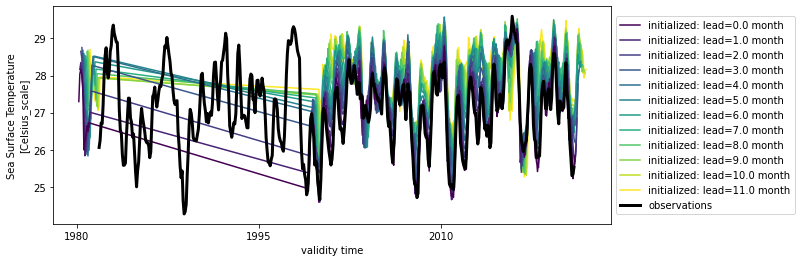```hindcast.verify(**metric_kwargs)[v].plot(label="no bias correction")
train_test_split = ["unfair", "unfair-cv", "fair"]

hindcast.remove_bias(
).verify(**metric_kwargs)[v].plot(label="unfair")

hindcast.remove_bias(
alignment=metric_kwargs["alignment"],
train_test_split="unfair-cv",
cv="LOO",
).verify(**metric_kwargs)[v].plot(label="unfair-cv")

hindcast.remove_bias(
alignment=metric_kwargs["alignment"],
train_test_split="fair",
train_time=slice("1982", "1998"),
).verify(**metric_kwargs)[v].plot(label="fair")

plt.legend()
plt.title(
f"NMME {hindcast.coords['model'].values} Nino3.4 SST {metric_kwargs['metric'].upper()}"
)
```
```Text(0.5, 1.0, 'NMME GFDL-CM2p5-FLOR-A06 Nino3.4 SST RMSE')
```# Comparison of methods `how`#

```methods = [
# "multiplicative_std",
"DetrendedQuantileMapping",
"EmpiricalQuantileMapping",
# "PrincipalComponents",
# "LOCI",
"QuantileDeltaMapping",
"Scaling",
"modified_quantile",
"basic_quantile",
# "gamma_mapping",
# "normal_mapping",
]
```
```import warnings

warnings.simplefilter("ignore")
```
```# xclim.sdba requires pint units
hindcast._datasets["initialized"][v].attrs["units"] = "C"
hindcast._datasets["observations"][v].attrs["units"] = "C"
```
```hindcast.sel(init=slice("1983", "2018")).verify(**metric_kwargs)[v].plot(label="raw")
for method in methods:
hindcast.remove_bias(
how=method, alignment=metric_kwargs["alignment"], train_test_split="unfair"
).verify(**metric_kwargs)[v].plot(label=method)
plt.legend()
```
```<matplotlib.legend.Legend at 0x142093d60>
```